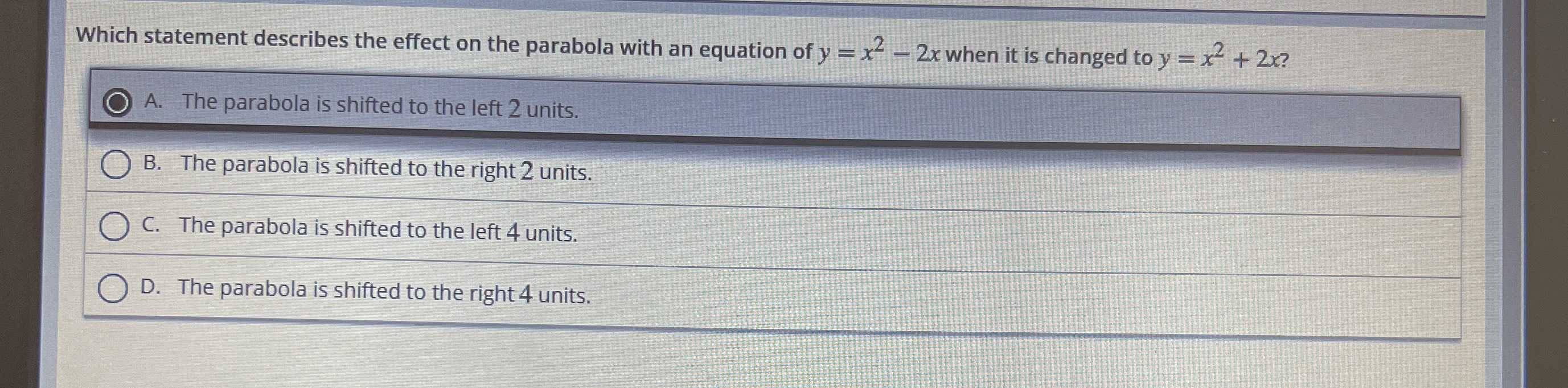### Still have math questions?

Algebra
QuestionWhich statement describes the effect on the parabola with an equation of $$y = x ^ { 2 } - 2 x$$ when it is changed to $$y = x ^ { 2 } + 2 x ?$$

A. The parabola is shifted to the left $$2$$ units.

B. The parabola is shifted to the right $$2$$ units.

C. The parabola is shifted to the left $$4$$ units.

D. The parabola is shifted to the right $$4$$ units.

A. The parabola is shifted to the left $$2$$ units.